Download the eSaral app and start learning from Kota's top IITians and doctors.

# Alcohol & Ether - JEE Main Previous Year Questions with Solutions

JEE Main Previous Year Papers Questions of Chemistry with Solutions are available at eSaral. Practicing JEE Mains chapter wise questions of Chemistry will help the JEE aspirants in realizing the question pattern as well as help in analyzing weak & strong areas. Simulator Previous Years AIEEE/JEE Mains Questions
Q. The number of stereoisomers possible for a compound of the molecular formula (1) 4        (2) 6         (3) 3           (4) 2 AIEEE-2009
Ans. (1)
Q. A liquid was mixed with ethanol and a drop of concentrated $\mathrm{H}_{2} \mathrm{SO}_{4}$ was added. A compound with a fruity smell was formed. The liquid was :- (1) $\mathrm{CH}_{3} \mathrm{COCH}_{3}$ (2) $\mathrm{CH}_{3} \mathrm{COOH}$ (3) $\mathrm{CH}_{3} \mathrm{OH}$ (4) HCHO AIEEE-2009
Ans. (2)
Q. From amongst the following alcohols the one that would react fastest with conc. HCl and anhydrous $\mathrm{ZnCl}_{2}$, is :- (1) 1–Butanol (2) 2–Butanol (3) 2–Methylpropan–2–ol (4) 2–Methylpropanol AIEEE-2010
Ans. (3)
Q. Consider the following reaction : $\mathrm{C}_{2} \mathrm{H}_{5} \mathrm{OH}+\mathrm{H}_{2} \mathrm{SO}_{4} \rightarrow$ Produce Among the following, which one cannot be formed as a product under any conditions ? (1) Ethyl-hydrogen sulphate (2) Ethylene (3) Acetylene (3) Diethyl ethe AIEEE-2011
Ans. (3)
Q. Iodoform can be prepared from all except :- (1) Isobutyl alcohol (2) Ethyl methyl ketone (3) Isopropyl alcohol (4) 3-Methyl–2–butanone AIEEE-2012
Ans. (1)
Q. An unknown alcohol is treated with the "Lucas reagent' to determine whether the alcohol is primary, secondary or tertiary. Which alcohol reacts fastest and by what mechanism :- (1) secondary alcoholby SN $^{1}$ (2) tertiary alcohol by SN $^{1}$ (3) secondary alcoholby SN $^{2}$ (4) tertiary alcohol by SN $^{2}$ AIEEE-2013
Ans. (2)
Q. The most suitable reagent for the conversion of $\mathrm{R}-\mathrm{CH}_{2}-\mathrm{OH} \rightarrow \mathrm{R}-\mathrm{CHO}$ is : (1) $\mathrm{CiO}_{3}$ (2) PCC (Pyridinium chlorochromate) (3) KMNO $_{4}$ (4) $\mathrm{K}_{2} \mathrm{Cr}_{2} \mathrm{O}_{7}$ Jee-Main-2014
Ans. (2)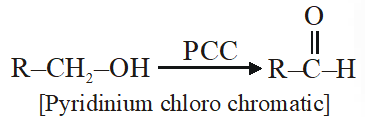Q. Allyl phenyl ether can be prepared by heating: (1) $\mathrm{CH}_{2}=\mathrm{CH}-\mathrm{CH}_{2}-\mathrm{Br}+\mathrm{C}_{6} \mathrm{H}_{5} \mathrm{ONa}$ (2) $\mathrm{C}_{6} \mathrm{H}_{5}-\mathrm{CH}=\mathrm{CH}-\mathrm{Br}+\mathrm{CH}_{3}-\mathrm{ONa}$ (3) $\mathrm{C}_{6} \mathrm{H}_{5} \mathrm{Br}+\mathrm{CH}_{2}=\mathrm{CH}-\mathrm{CH}_{2}-\mathrm{ONa}$ (4) $\mathrm{CH}_{2}=\mathrm{CH}-\mathrm{Br}+\mathrm{C}_{6} \mathrm{H}_{5}-\mathrm{CH}_{2}-\mathrm{ONa}$ Jee-Main-2014
Ans. (1)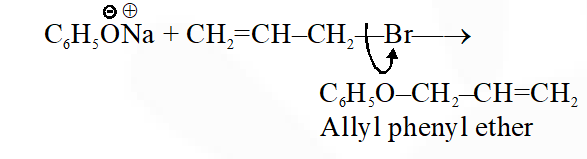Q. In the Victor-Meyer's test, the colour given by $1^{\circ}, 2^{\circ}$ and $3^{\circ}$ alcohols are respectively :- (1) Red, blue, colourless (2) Colourless, red, blue (3) Red, blue, violet (4) Red, colourless, blue Jee-Main-2014
Ans. (1)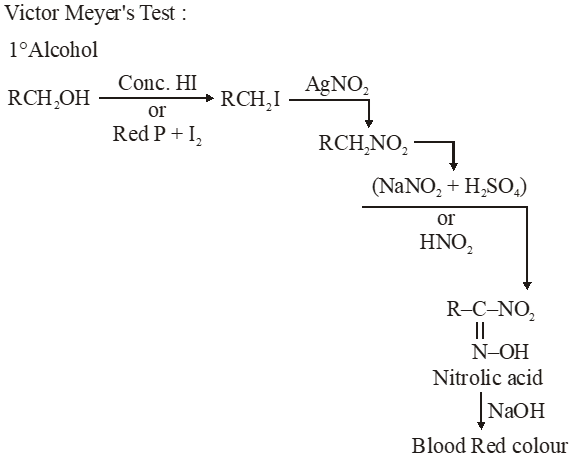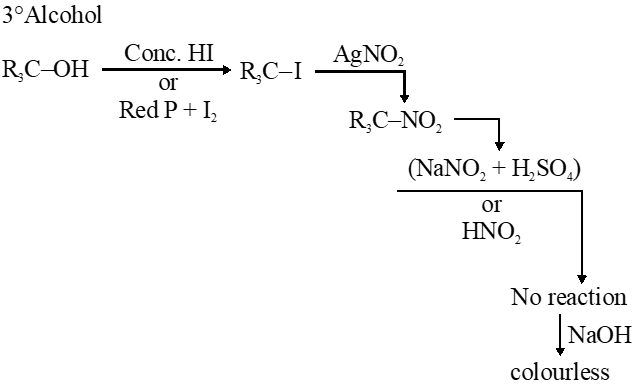Q. Williamson synthesis of ether is an example of (1) Nucleophilic addition (2) Electrophilic substitution (3) Nucleophilic substitution (4) Electrophilic addition Jee-Main-2014
Ans. (3) Nucleophilic substitution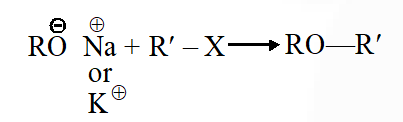Q. Phenol on treatment with $\mathrm{CO}_{2}$ in the presence of NaOH followed by acidification produces compound X as the major product. X on treatment with $\left(\mathrm{CH}_{3} \mathrm{CO}\right)_{2} \mathrm{O}$ in the presence of catalytic amount of $\mathrm{H}_{2} \mathrm{SO}_{4}$ produces :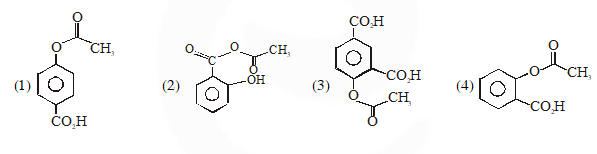Jee-Main-2018
Ans. (4)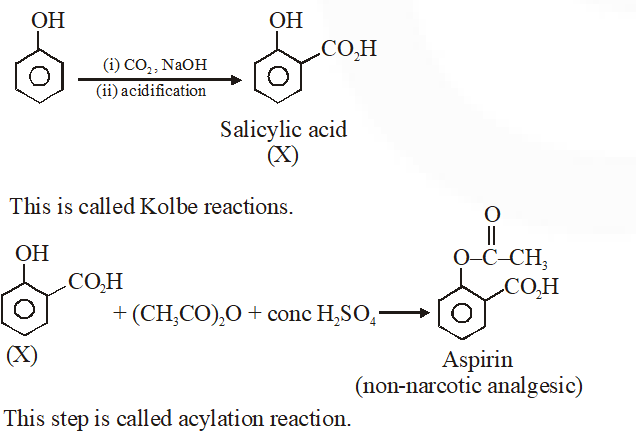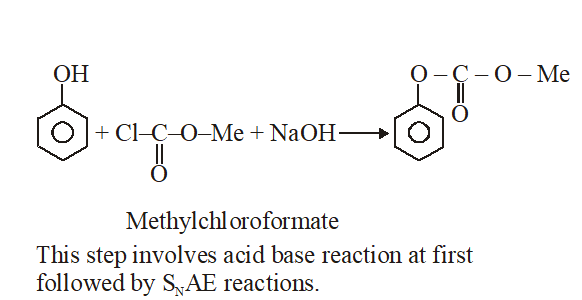Q. Phenol reacts with methyl chloroformate in the presence of NaOH to form product A. A reacts with $\mathrm{Br}_{2}$ to form product B. A and B are respectively :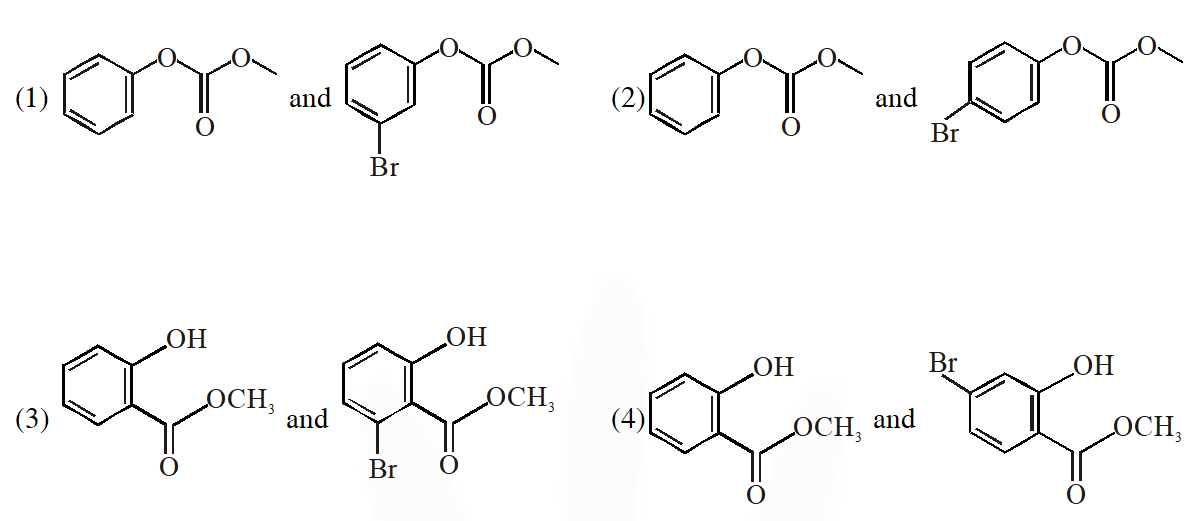Jee-Main-2018
Ans. (2)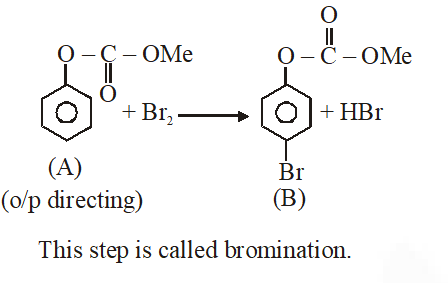Shreyas
March 19, 2023, 6:35 a.m.
Thank you so much for this JEE questions 🙏
THIS COMMENT HAS 20 LIKES
April 8, 2021, 5 p.m.
THIS COMMENT HAS 20 LIKES
uhdeoideo
Jan. 23, 2021, 10:14 p.m.
abe ye log kam questions dete h aur bacho ko bewakoof banate h
ambika
Dec. 22, 2020, 8:22 p.m.
tqsm.....
ambika
Dec. 22, 2020, 8:21 p.m.
tqsm.....😊
ipta Dash
Sept. 3, 2020, 5:28 p.m.
bht bhala
Vedansh vashi
Aug. 26, 2020, 8:06 a.m.
Very useful. Thank you very much
chudail'
Aug. 20, 2020, 8 p.m.
higher level questions......
Uma
Aug. 18, 2020, 11:44 a.m.
Thanks very useful
Arjun
Aug. 7, 2020, 10:37 a.m.
Helpful but if you give answer with explanation....then it would be great help
pankaj
July 15, 2020, 10:55 a.m.
its nice
Royal
June 7, 2020, 12:37 p.m.